# Java Program to Count number of Digits In Number

In this tutorial you will learn about the Java Program to Count number of Digits In Number and its application with practical example.

## Java Program to Count Number of Digits In Number

In this tutorial, we will learn to create a Java program that will count the number of digits in number in Java programming.

## Prerequisites

Before starting with this tutorial, we assume that you are the best aware of the following Java programming topics:

• Operators in Java Programming.
• Basic Input and Output function in Java Programming.
• Basic Java programming.
• While Loop in Java programming.

## Count the number of digits in the number.

In today’s tutorial, we will learn to create a java program that will count the number of digits of the number. First, we will take the number in input from the user. Then we will pass that number to the while loop to count digits. At last, we will print the number of digits in the number.

With the help of this program, we can count the number of digits in numbers.

## Output:-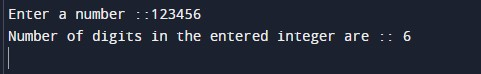In the above program, we have first initialized the required variable.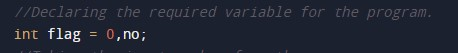• flag  = it will hold the integer value.
• digits = it will hold the integer value for numbers.
• no = it will hold the integer value of number input.

Taking input number from the user.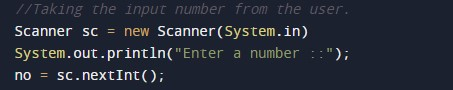Counting the digits of the number.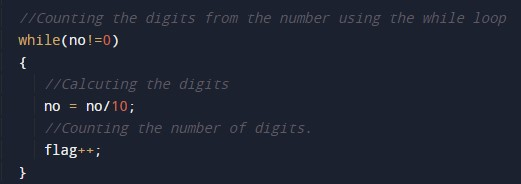Printing the numbers of digits from the program.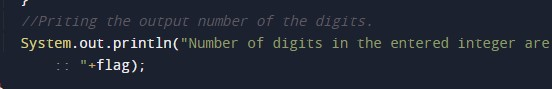In this tutorial we have learn about the Java Program to Count number of Digits In Number and its application with practical example. I hope you will like this tutorial.